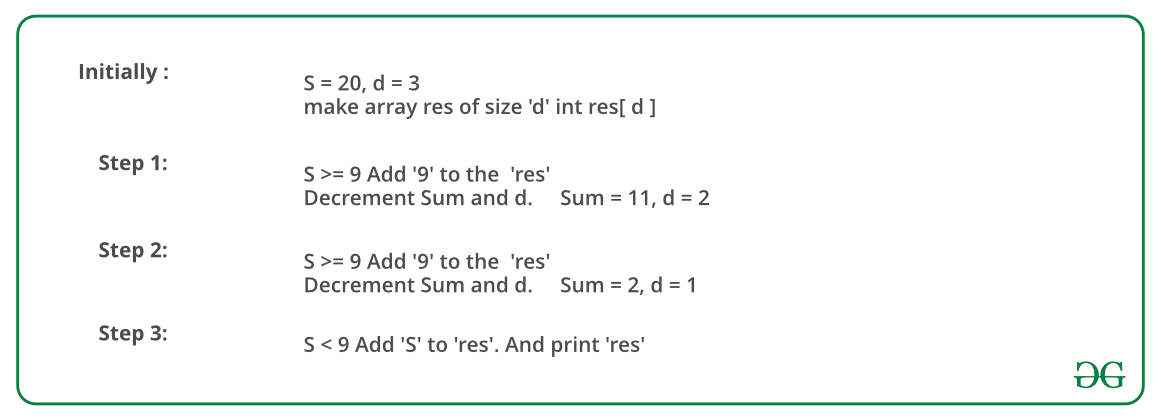Related Articles

# Find the Largest number with given number of digits and sum of digits

• Difficulty Level : Easy
• Last Updated : 29 Jun, 2021

How to find the largest number with given digit sum s and number of digits d?
Examples:

```Input  : s = 9, d = 2
Output : 90

Input  : s = 20, d = 3
Output : 992```

A Simple Solution is to consider all m digit numbers and keep track of maximum number with digit sum as s. A close upper bound on time complexity of this solution is O(10m).
There is a Greedy approach to solve the problem. The idea is to one by one fill all digits from leftmost to rightmost (or from most significant digit to least significant).
We compare the remaining sum with 9 if the remaining sum is more than 9, we put 9 at the current position, else we put the remaining sum. Since we fill digits from left to right, we put the highest digits on the left side, hence get the largest number.
Below image is an illustration of the above approach:Below is the implementation of the above approach:

## C++

 `// C++ program to find the largest number that can be``// formed from given sum of digits and number of digits.``#include ``using` `namespace` `std;` `// Prints the smalles possible number with digit sum 's'``// and 'm' number of digits.``void` `findLargest(``int` `m, ``int` `s)``{``    ``// If sum of digits is 0, then a number is possible``    ``// only if number of digits is 1.``    ``if` `(s == 0)``    ``{``        ``(m == 1)? cout << ``"Largest number is "` `<< 0``                       ``: cout << ``"Not possible"``;``        ``return` `;``    ``}` `    ``// Sum greater than the maximum possible sum.``    ``if` `(s > 9*m)``    ``{``        ``cout << ``"Not possible"``;``        ``return` `;``    ``}` `    ``// Create an array to store digits of result``    ``int` `res[m];` `    ``// Fill from most significant digit to least``    ``// significant digit.``    ``for` `(``int` `i=0; i= 9)``        ``{``            ``res[i] = 9;``            ``s -= 9;``        ``}` `        ``// If remaining sum becomes less than 9, then``        ``// fill the remaining sum``        ``else``        ``{``            ``res[i] = s;``            ``s = 0;``        ``}``    ``}` `    ``cout << ``"Largest number is "``;``    ``for` `(``int` `i=0; i

## Java

 `// Java program to find the largest number that can be``// formed from given sum of digits and number of digits` `class` `GFG``{``    ``// Function to print the largest possible number with digit sum 's'``    ``// and 'm' number of digits``    ``static` `void` `findLargest(``int` `m, ``int` `s)``    ``{``        ``// If sum of digits is 0, then a number is possible``        ``// only if number of digits is 1``        ``if` `(s == ``0``)``        ``{``            ``System.out.print(m == ``1` `? ``"Largest number is 0"` `: ``"Not possible"``);``            ` `            ``return` `;``        ``}`` ` `        ``// Sum greater than the maximum possible sum``        ``if` `(s > ``9``*m)``        ``{``            ``System.out.println(``"Not possible"``);``            ``return` `;``        ``}`` ` `        ``// Create an array to store digits of result``        ``int``[] res = ``new` `int``[m];`` ` `        ``// Fill from most significant digit to least``        ``// significant digit``        ``for` `(``int` `i=``0``; i= ``9``)``            ``{``                ``res[i] = ``9``;``                ``s -= ``9``;``            ``}`` ` `            ``// If remaining sum becomes less than 9, then``            ``// fill the remaining sum``            ``else``            ``{``                ``res[i] = s;``                ``s = ``0``;``            ``}``        ``}`` ` `        ``System.out.print(``"Largest number is "``);``        ``for` `(``int` `i=``0``; i

## Python3

 `# Python 3 program to find``# the largest number that``# can be formed from given``# sum of digits and number``# of digits.`  `# Prints the smalles``# possible number with digit``# sum 's' and 'm' number of``# digits.``def` `findLargest( m, s) :` `    ``# If sum of digits is 0,``    ``# then a number is possible``    ``# only if number of digits``    ``# is 1.``    ``if` `(s ``=``=` `0``) :``    ` `        ``if``(m ``=``=` `1``) :``            ``print``(``"Largest number is "` `, ``"0"``,end ``=` `"")``        ``else` `:``            ``print``(``"Not possible"``,end ``=` `"")``    ` `        ``return` `    ``# Sum greater than the``    ``# maximum possible sum.``    ``if` `(s > ``9` `*` `m) :``        ``print``(``"Not possible"``,end ``=` `"")``        ``return``    ` `    ``# Create an array to``    ``# store digits of``    ``# result``    ``res ``=` `[``0``] ``*` `m` `    ``# Fill from most significant``    ``# digit to least significant``    ``# digit.``    ``for` `i ``in` `range``(``0``, m) :``        ` `        ``# Fill 9 first to make``        ``# the number largest``        ``if` `(s >``=` `9``) :``            ``res[i] ``=` `9``            ``s ``=` `s ``-` `9``        ` `        ``# If remaining sum``        ``# becomes less than``        ``# 9, then fill the``        ``# remaining sum``        ``else` `:``            ``res[i] ``=` `s``            ``s ``=` `0``        ` `        ` `    ``print``( ``"Largest number is "``,end ``=` `"")``    ` `    ``for` `i ``in` `range``(``0``, m) :``        ``print``(res[i],end ``=` `"")` `# Driver code``s ``=` `9``m ``=` `2``findLargest(m, s)` `# This code is contributed by Nikita Tiwari.`

## C#

 `// C# program to find the``// largest number that can``// be formed from given sum``// of digits and number of digits``using` `System;` `class` `GFG``{``    ` `    ``// Function to print the ``    ``// largest possible number``    ``// with digit sum 's' and``    ``// 'm' number of digits``    ``static` `void` `findLargest(``int` `m, ``int` `s)``    ``{``        ``// If sum of digits is 0,``        ``// then a number is possible``        ``// only if number of digits is 1``        ``if` `(s == 0)``        ``{``            ``Console.Write(m == 1 ?``                   ``"Largest number is 0"` `:``                          ``"Not possible"``);``            ` `            ``return` `;``        ``}` `        ``// Sum greater than the``        ``// maximum possible sum``        ``if` `(s > 9 * m)``        ``{``            ``Console.WriteLine(``"Not possible"``);``            ``return` `;``        ``}` `        ``// Create an array to``        ``// store digits of result``        ``int` `[]res = ``new` `int``[m];` `        ``// Fill from most significant``        ``// digit to least significant digit``        ``for` `(``int` `i = 0; i < m; i++)``        ``{``            ``// Fill 9 first to make``            ``// the number largest``            ``if` `(s >= 9)``            ``{``                ``res[i] = 9;``                ``s -= 9;``            ``}` `            ``// If remaining sum becomes``            ``// less than 9, then``            ``// fill the remaining sum``            ``else``            ``{``                ``res[i] = s;``                ``s = 0;``            ``}``        ``}` `        ``Console.Write(``"Largest number is "``);``        ``for` `(``int` `i = 0; i < m; i++)``            ``Console.Write(res[i]);``    ``}``    ` `    ``// Driver Code``    ``static` `public` `void` `Main ()``    ``{``        ``int` `s = 9, m = 2;``        ``findLargest(m, s);``    ``}``}` `// This code is Contributed by ajit`

## PHP

 ` 9 * ``\$m``)``    ``{``        ``echo` `"Not possible"``;``        ``return` `;``    ``}` `    ``// Create an array to store``    ``// digits of result Fill from``    ``// most significant digit to``    ``// least significant digit.``    ``for` `(``\$i` `= 0; ``\$i` `< ``\$m``; ``\$i``++)``    ``{``        ``// Fill 9 first to make``        ``// the number largest``        ``if` `(``\$s` `>= 9)``        ``{``            ``\$res``[``\$i``] = 9;``            ``\$s` `-= 9;``        ``}` `        ``// If remaining sum becomes``        ``// less than 9, then fill``        ``// the remaining sum``        ``else``        ``{``            ``\$res``[``\$i``] = ``\$s``;``            ``\$s` `= 0;``        ``}``    ``}` `    ``echo` `"Largest number is "``;``    ``for` `(``\$i` `= 0; ``\$i` `< ``\$m``; ``\$i``++)``        ``echo` `\$res``[``\$i``];``}` `// Driver code``\$s` `= 9; ``\$m` `= 2;``findLargest(``\$m``, ``\$s``);` `// This code is contributed by m_kit``?>`

## Javascript

 ``

Output :

`Largest number is 90`

Time Complexity of this solution is O(m).
This article is contributed by Vaibhav Agarwal. If you like GeeksforGeeks and would like to contribute, you can also write an article and mail your article to review-team@geeksforgeeks.org. See your article appearing on the GeeksforGeeks main page and help other Geeks.